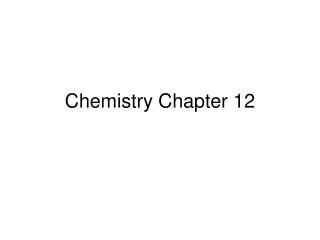Download PresentationChemistry Chapter 12

# Chemistry Chapter 12

Download Presentation## Chemistry Chapter 12

- - - - - - - - - - - - - - - - - - - - - - - - - - - E N D - - - - - - - - - - - - - - - - - - - - - - - - - - -
##### Presentation Transcript

1. Chemistry Chapter 12

2. stoichiometry. • that portion of chemistry dealing with numerical relationships in chemical reactions; the calculation of quantities of substances involved in chemical equations

3. A balanced chemical equation can be interpreted in terms of different quantities, including numbers of atoms, molecules, or moles; mass; and volume.

4. Mass and atoms are conserved in every chemical reaction.

5. Number of Atoms • At the atomic level, a balanced equation indicates that the number and type of each atom that makes up each reactant also makes up each product.

6. Number of Molecules • The balanced equation indicates that one molÂecule of nitrogen reacts with three molecules of hydrogen. Nitrogen and hydrogen will always react to form ammonia in a 1:3:2 ratio of molecules.

7. Moles • A balanced chemical equation also tells you the number of moles of reactants and products. The coefficients of a balanced chemical equation indicate the relative numbers of moles of reactants and products in a chemical reaction

8. Mass • A balanced chemical equation obeys the law of conservation of mass. This law states that mass can be neither created nor destroyed in an ordinary chemical or physical process.

9. Volume • If you assume standard temperature and pressure, the equation also tells you about the volumes of gases. Recall that 1 mol of any gas at STP occupies a volume of 22.4 L.

10. However, molecules, formula units, moles, and volumes are not necessarily conserved—although they may be. Consider, for example, the formation of hydrogen iodide,

11. (5) Key Concept How is a balanced equation similar to a recipe?Hint • (6) Key Concept How do chemists use balanced equations?Hint • (7) Key Concept Chemical reactions can be described in terms of what quantities?Hint • (8) Key Concept What quantities are always conserved in chemical reactions?Hint

12. (9)Interpret the given equation in terms of relative numbers of representative particles, numbers of moles, and masses of reactants and products. • 2K (s) + 2H2O (l) → 2KOH (aq) + H2 (g)

13. (10)Balance this equation: C2H5OH(l) + O2 (g) → CO2 (g) + H2O(g). Show that the balanced equation obeys the law of conservation of mass.

14. mole ratio • a conversion factor derived from the coefficients of a balanced chemical equation interpreted in terms of moles

15. In chemical calculations, mole ratios are used to convert between moles of reactant and moles of product, between moles of reactants, or between moles of products.

16. When multiplying and dividing measurements, the rounded answer can have no more significant figures than the least number of significant figures in any measurement in the calculation.

17. When adding and subtracting measurements, the answer can have no more decimal places than the least number of decimal places in any measurement in the problem. The difference of 8.78 cm − 2.2 cm = 6.58 cm is rounded to 6.6 cm (one decimal place).

18. In a typical stoichiometric problem, the given quantity is first converted to moles. Then the mole ratio from the balanced equation is used to calculate the number of moles of the wanted substance. Finally, the moles are converted to any other unit of measurement related to the unit mole, as the problem requires.

19. Recall from Chapter 10 that the mole can be related to other quantities as well. For example, 1 mol = 6.02 × 10 23 representative particles, and 1 mol of a gas = 22.4 L at STP. These two relationships provide four more conversion factors that you can use in stoichiometric calculations.